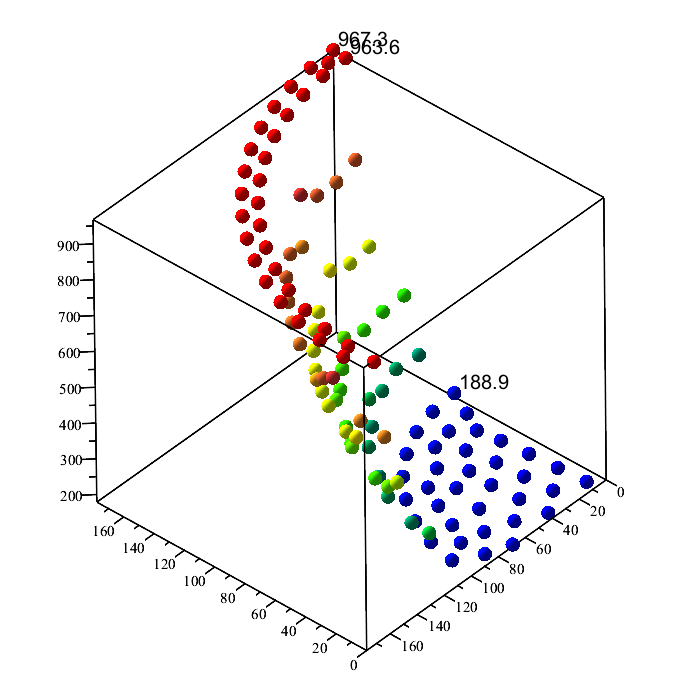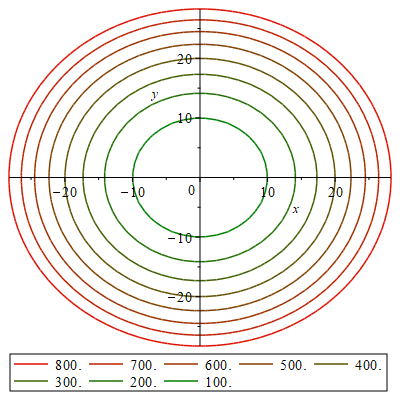# Question:How to plot Iso-Lines from a set of values with coordinates

## Question:How to plot Iso-Lines from a set of values with coordinates

Maple 2021

Hi,

I am struggling with a task of creating a 2D contourplot of temperature values showing Iso-Lines (lines of equal temperatures in steps of 100°C).

I have a set of temperature values with corresponding x and y coordinates of a cross section. The data is given as an unsorted set of X-Y-Temperature data:

[[177.80000,0.,967.3266667],[169.80000,0.,963.5900000],[100.00000,0.,188.8933333],[176.92000,17.703000,967.3266667],[174.27000,35.230000,967.3200000],[169.90000,52.407000,967.3566667],[163.84000,69.064000,967.3366667],[156.15000,85.034000,967.3100000],[146.91000,100.16000,967.3366667],[136.20000,114.29000,967.3666667],...]

I can plot the data as a 3D pointplot and assign colors based on the temperature value:

plots:-pointplot3d(<ThermRes(2 .. (), 2) | ThermRes(2 .. (), 3) | ThermRes(2 .. (), TimeCol)>, colorscheme = ["zgradient", ["blue", "green", "yellow", "orange", "red"]], style = point, symbol = solidsphere, symbolsize = 20)For better visualization, I would like to plot this data in a 2D contourplot showing coloured Iso-lines in 100°C steps, similar to the following scheme:I know this is not directly possible from xyz datapoints. Therefore, I was trying to create an interpolation function using the Interpolation package. I have tried to find out if it is possible to get a working interpolation function for my data, but it seems like the x and y coordinates must be always in increasing order and there has to be a z value (temperature data point) for each pair of coordinates in matrix form, is that correct? Unfortunately, as you can see above, my x-y coordinate pairs are irregular and unsorted and I do not have a temperature value for every combination of x and y coordinate values.

Is there any way how to find an interpolation function for this kind of data that would enable a contourplot? Or any other ideas how to create a coloured 2D plot from this data with a legend showing the 100°C temperature color values?

Greetings, Oliver﻿